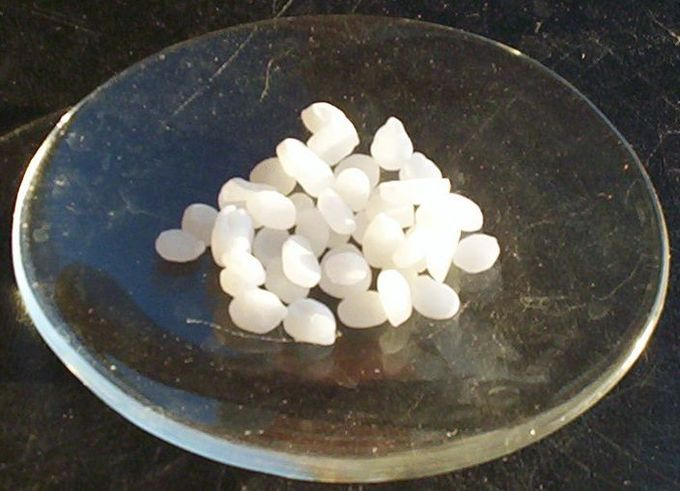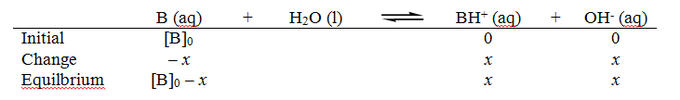## Strong Bases

Strong bases either dissociate completely in solution to yield hydroxide ions, or deprotonate water to yield hydroxide ions.

### Learning Objectives

Recognize strong bases and their chemical properties.

### Key Takeaways

#### Key Points

• In chemistry, a base is a substance that can either accept hydrogen ions (protons) or, more generally, donate a pair of valence electrons; it can be thought of as the chemical opposite of an acid.
• Strong bases are commonly, though not exclusively, formed from the hydroxides of alkali metals and alkaline earth metals.
• Superbases are stronger than hydroxide ions and cannot be kept in water; they provide examples of bases that do not contain a hydroxide ion (and are therefore strong Lewis and/or Bronsted-Lowry bases, but not Arrhenius bases).

#### Key Terms

• base: a proton acceptor, or an electron pair donor
• solvate: a complex formed from solvent molecules attaching to a solute
• dissociation: the process by which compounds split into smaller constituent molecules, usually reversibly

As discussed in the previous concepts on bases, a base is a substance that can: donate hydroxide ions in solution (Arrhenius definition); accept H+ ions (protons) (Bronsted-Lowry definition); or donate a pair of valence electrons (Lewis definition). In water, basic solutions have a pH higher than 7.0, indicating a greater concentration of OH than H+.

### Strong Arrhenius Bases

A strong Arrhenius base, like a strong acid, is a compound that ionizes completely or near-completely in solution. Therefore, the concentration of hydroxide ions in a strongly basic solution is equal to that of the undissociated base. Common examples of strong Arrhenius bases are the hydroxides of alkali metals and alkaline earth metals such as NaOH and Ca(OH)2. Strong bases are capable of deprotonating weak acids; very strong bases can deprotonate very weakly acidic C–H groups in the absence of water.Sodium hydroxide pellets: Sodium hydroxide pellets, before being suspended in water to dissociate.

Some common strong Arrhenius bases include:

• Potassium hydroxide (KOH)
• Sodium hydroxide (NaOH)
• Barium hydroxide (Ba(OH)2)
• Caesium hydroxide (CsOH)
• Strontium hydroxide (Sr(OH)2)
• Calcium hydroxide (Ca(OH)2)
• Lithium hydroxide (LiOH)
• Rubidium hydroxide (RbOH)

The cations of these strong bases appear in the first and second groups of the periodic table (alkali and earth alkali metals). Generally, the alkali metal bases are stronger than the alkaline earth metal bases, which are less soluble. When writing out the dissociation equation of a strong base, assume that the reverse reaction does not occur, because the conjugate acid of a strong base is very weak.

### Superbases (Lewis bases)

Group 1 salts of carbanions (such as butyllithium, LiC4H9, which dissociates into Li+ and the carbanion C4H9), amides (NH2), and hydrides (H) tend to be even stronger bases due to the extreme weakness of their conjugate acids—stable hydrocarbons, amines, and hydrogen gas. Usually, these bases are created by adding pure alkali metals in their neutral state, such as sodium, to the conjugate acid. They are called superbases, because it is not possible to keep them in aqueous solution; this is due to the fact they will react completely with water, deprotonating it to the fullest extent possible. For example, the ethoxide ion (conjugate base of ethanol) will undergo this reaction in the presence of water:

CH3CH2O + H2O → CH3CH2OH + OH

Unlike weak bases, which exist in equilibrium with their conjugate acids, the strong base reacts completely with water, and none of the original anion remains after the base is added to solution. Some other superbases include:

• Butyl lithium (n-BuLi)
• Lithium diisopropylamide (LDA) (C6H14LiN)
• Lithium diethylamide (LDEA)
• Sodium amide (NaNH2)
• Sodium hydride (NaH)
• Lithium bis(trimethylsilyl)amide, ((CH3)3Si)2NLi

Superbases such as the ones listed above are commonly used as reagents in organic laboratories.

## Weak Bases

In aqueous solution, a weak base reacts incompletely with water to yield hydroxide ions.

### Learning Objectives

Solve acid-base equilibrium problems involving weak bases.

### Key Takeaways

#### Key Points

• A base is a substance that can accept hydrogen ions (H+) or, more generally, donate a pair of valence electrons; a weak base does not, therefore, fully ionize or completely accept hydrogen ions in an aqueous solution.
• Bases increase pH; weak bases have a less dramatic effect on pH.
• pOH is occasionally used as an alternative to pH to quantify the relative H+/hydroxide concentration in solution.
• A base dissociation constant, Kb, mathematically represents the base’s relative strength and is analogous to the acid dissociation constant; weaker bases have smaller Kb values.
• Like weak acids, weak bases can be used to make buffer solutions.

#### Key Terms

• weak base: a proton acceptor that does not ionize fully in an aqueous solution
• enol: an organic alcohol with an -OH functional group located off a double bond
• enolate: a deprotonated enol

A base is a substance that can accept hydrogen ions (H+) or, more generally, donate a pair of valence electrons. A weak base is a chemical base that does not ionize fully in an aqueous solution. As Brønsted-Lowry bases are proton acceptors, a weak base may also be defined as a chemical base with incomplete protonation. A general formula for base behavior is as follows:

$\text{B}(\text{aq}) + \text{H}_2\text{O}(\text{aq}) \rightleftharpoons \text{BH}^+(\text{aq}) + \text{OH}^-(\text{aq})$

A base can either accept protons from water molecules or donate hydroxide ions to a solution. Both actions raise the pH of the solution by decreasing the concentration of H+ ions. This results in a relatively low pH compared to that of strong bases. The pH of bases in aqueous solution ranges from greater than 7 (the pH of pure water) to 14 (though some bases have pH values greater than 14). The formula for pH is:

pH = -log10[H+]

Sometimes, however, it is more convenient to focus on the pOH of bases, rather than the pH. The pOH more directly references the [OH].

pOH = -log10[OH]

Some common weak bases and their corresponding pKb values include:

• C6H5NH2 (9.38)
• NH3 (4.75)
• CH3NH2 (3.36)
• CH3CH2NH2 (3.27)

Smaller pKb values indicate higher values of Kb; this also indicates a stronger base.

Like weak acids, weak bases have important applications in biochemical studies, chemistry reactions, and physiological purposes, particularly because of their role in buffer solutions. Weak bases can also be used to catalyze certain reactions, such as enolate formation, as demonstrated in the figure below:Weak base catalyzing enolate formation: A weak base, symbolized by B:, can catalyze enolate formation by acting as a proton acceptor.

## Base Dissociation Constant

The base dissociation constant (Kb) measures a base’s relative strength.

### Learning Objectives

Calculate the Kw (water dissociation constant) using the following equation: Kw = [H+] x [OH−]  and manpulate the formula to determine [OH−] = Kw/[H+] or [H+]=Kw/[OH-]

### Key Takeaways

#### Key Points

• The base dissociation constant KbE measures a base’s basicity, or strength.
• Kb is related to the acid dissociation constant, Ka, by the simple relationship pKa + pKb = 14, where pKb and pKa are the negative logarithms of Kb and Ka, respectively.
• Kb and Ka are also related through the ion constant for water, Kw, by the relationship $\text{K}_\text{W}=\text{K}_\text{a}\times \text{K}_\text{b}$.

#### Key Terms

• conjugate acid: the species created when a base accepts a proton

In chemistry, a base is a substance that can accept hydrogen ions (protons) or, more generally, donate a pair of valence electrons. The base dissociation constant, Kb, is a measure of basicity—the base’s general strength. It is related to the acid dissociation constant, Ka, by the simple relationship pKa + pKb = 14, where pKb and pKa are the negative logarithms of Kb and Ka, respectively. The base dissociation constant can be expressed as follows:

$\text{K}_\text{b} = \dfrac{[\text{BH}^+][\text{OH}^-]}{\text{B}}$

where $\text{B}$ is the base, $\text{BH}^+$ is its conjugate acid, and $\text{OH}^-$ is hydroxide ions.

### The Base Dissociation Constant

Historically, the equilibrium constant Kb for a base has been defined as the association constant for protonation of the base, B, to form the conjugate acid, HB+.

$\text{B}(\text{aq}) + \text{H}_2\text{O}(\text{l}) \leftrightharpoons \text{HB}^+(\text{aq}) + \text{OH}^-(\text{aq})$

As with any equilibrium constant for a reversible reaction, the expression for Kb takes the following form:

$\text{K}_{\text{b}} = \frac{[\text{OH}^{-}][\text{HB}^{+}]}{[\text{B}]}$

Kb is related to Ka for the conjugate acid. Recall that in water, the concentration of the hydroxide ion, [OH], is related to the concentration of the hydrogen ion by the autoionization constant of water:

$\text{K}_\text{W}=[\text{H}^+][\text{OH}^-]$

Rearranging, we have:

$[\text{OH}^{-}] = \frac{\text{K}_{\text{w}}}{[\text{H}^{+}]}$

Substituting this expression for [OH] into the expression for Kb yields:

$\text{K}_{\text{b}} = \frac{\text{K}_{\text{w}}[\text{HB}^{+}]}{[\text{B}][\text{H}^{+}]} = \frac{\text{K}_{\text{w}}}{\text{K}_{\text{a}}}$

Therefore, for any base/conjugate acid pair, the following relationship always holds true:

$\text{K}_\text{W}=\text{K}_\text{a}\text{K}_\text{b}$

Taking the negative log of both sides yields the following useful equation:

$\text{pK}_\text{a}+\text{pK}_\text{b}=14$

In actuality, there is no need to define pKb separately from pKa, but it is done here because pKb values are found in some of the older chemistry literature.

### Calculating the pH of a Weak Base in Aqueous Solution

The pH of a weak base in aqueous solution depends on the strength of the base (given by Kb) and the concentration of the base (the molarity, or moles of the base per liter of solution). A convenient way to find the pH for a weak base in solution is to use an ICE table: ICE stands for “Initial,” “Change,” and”Equilibrium.”

Before the reaction starts, the base, B, is present in its initial concentration [B]0, and the concentration of the products is zero. As the reaction reaches equilibrium, the base concentration decreases by x amount; given the reaction’s stoichiometry, the two products increase by x amount. At equilibrium, the base’s concentration is [B]0x, and the two products’ concentration is x.ICE diagram: An ICE diagram for a weak base in aqueous solution.

The Kb for the reaction is:

$\text{K}_{\text{b}} = \frac{[\text{BH}^+][\text{OH}^-]}{[\text{B}]}$

Filling in the values from the equilibrium line gives:

$\text{K}_{\text{b}} = \frac{\text{x}^2}{[\text{B}]_{0}-\text{x}}$

This quadratic equation can be solved for x. However, if the base is weak, then we can assume that x will be insignificant compared to [B]0, and the approximation [B]0x ≈ [B]0 can be used. The equation simplifies to:

$\text{K}_{\text{b}} = \frac{\text{x}^2}{[\text{B}]_{0}}$

Since x = [OH], we can calculate pOH using the equation pOH = –log[OH]; we can find the pH using the equation 14 – pOH = pH.